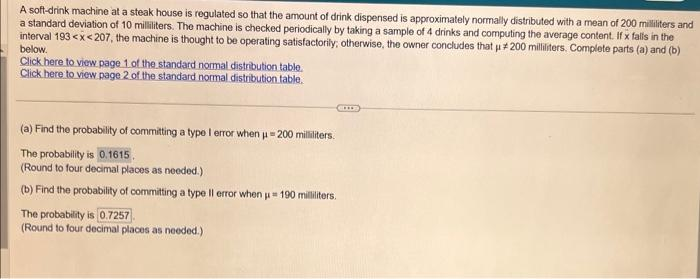Home / Expert Answers / Statistics and Probability / part-b-a-soft-drink-machine-at-a-steak-house-is-regulated-so-that-the-amount-of-drink-dispensed-is-a-pa957

# (Solved): part b A soft-drink machine at a steak house is regulated so that the amount of drink dispensed is a ...

part bA soft-drink machine at a steak house is regulated so that the amount of drink dispensed is approximately normally distributed with a mean of 200 mililiters and a standard deviation of 10 mililiters. The machine is checked periodically by taking a sample of 4 drinks and computing the average content. If $$x$$ falls in the interval $$193<\bar{x}<207$$, the machine is thought to be operating satisfactorily, otherwise, the owner concludes that $$\mu \neq 200$$ milliiters, Complete parts (a) and (b) below. Click here to view page 1 of the standard normal distribution table. Click here to view page 2 of the standard normal distribution table. (a) Find the probability of commiting a type I error when $$\mu=200$$ milliliters. The probability is $$0.1615$$, (Round to four decimal places as needed.) (b) Find the probability of committing a type $$\|$$ error when $$\mu=190$$ milliliters. The probability is (Round to four decimal places as needed.)

We have an Answer from Expert